### IRR Function Examples – Excel, VBA, & Google Sheets

This tutorial demonstrates how to use the Excel IRR Function in Excel to calculate the internal rate of return of a set of periodic cash flows.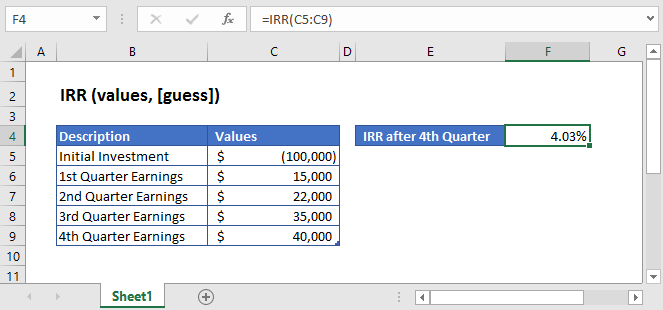## IRR Function Overview

The IRR Function Calculates the internal rate of return for a series of periodic (consistent timing) cash flows. If the cash flows are not periodic, use the XIRR function instead.

To use the IRR Excel Worksheet Function, select a cell and type: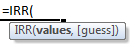(Notice how the formula inputs appear)

### IRR Function Syntax and Inputs:

values – The values argument consists of an array or a reference to a range of cells representing the series of cash flows. And the values argument must contain at least one negative and one positive value for the calculation.

guess – It’s an estimate of what the internal rate of return should be. If this argument is omitted then it assumes the default value of 0.1 or 10%.

AutoMacro - VBA Code Generator

## What Is Internal Rate of Return?

The internal Rate of Return is the interest rate or the discount rate that makes the Net Present Value (NPV) of an investment equals to zero. It is the expected rate of return that will be earned on investment under consideration. The calculated IRR needs to satisfy the following equation.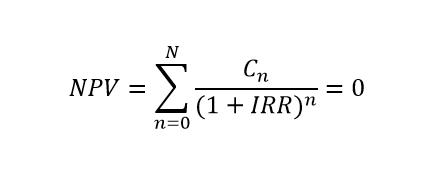Where:

Cn = Series of cash flows

IRR = Internal Rate of Return

n = Number of periods

## What Is Excel IRR Function?

IRR function calculates the internal rate of return for a series of cash flows occurring at regular intervals.

### Calculate Internal Rate of Return of an investment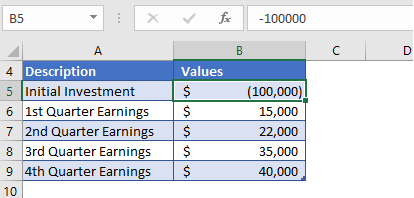For the initial investment of \$10,000, the earnings of the 1st, 2nd, 3rd, and 4th quarters are given in the above table.

Here, the initial investment is entered with a negative sign as its showing payment outgoing, and the quarterly earnings are represented by positive values.

Initial investment = -\$100,000

The formula used for the calculation is: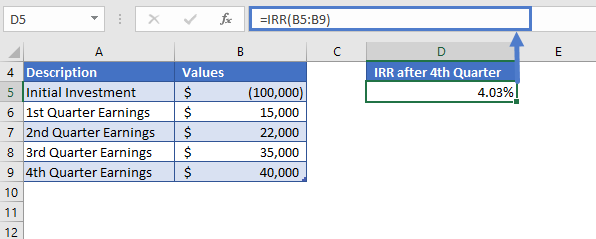The IRR function returns the value of

IRR = 4.03%.

### Example 2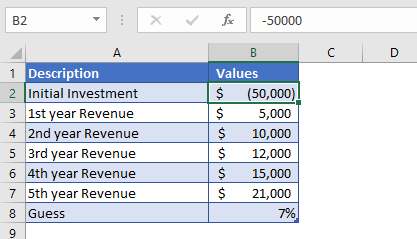Suppose, you are running a business for five years and want to figure out the rate of return for your cash flows. The initial investment for this business required was \$50,000 and the revenues generated through sales are given in the above table. Our initial guess for this is 7%.

The formula used for the calculation is: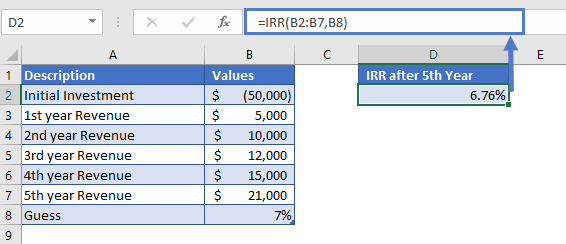The Internal Rate of Return we get from the IRR function is

IRR = 6.76%

This is very close to our guess.

## Additional Notes

Use the IRR Function to calculate the Internal Rate of Return of investment.

An iterative technique is used for calculating the IRR value. So, even after 20 tries and starting with the guess the IRR function can’t find a value that’s accurate within 0.00001%, it returns the #NUM! error.

If the IRR function returns #NUM! or unexpected results then try again with different guess values.

The values should in chronological order, meaning the sequence of payments and income should be entered correctly.

Periodic cash flows are required. Cash flows most occur on a consistent basis each period.

The result of the IRR Function may appear as a decimal. Change the cell Number Formatting to Percentage to display the function result as a percentage:## IRR in Google Sheets

All of the above examples work exactly the same in Google Sheets as in Excel.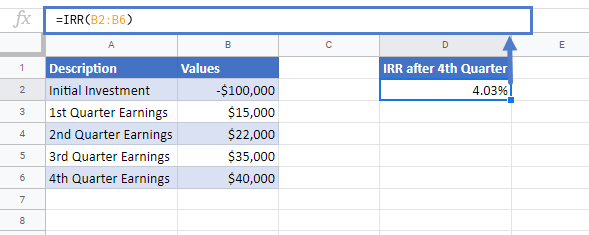## IRR Examples in VBA

You can also use the IRR function in VBA. Type:
`application.worksheetfunction.irr(values,guess)`
For the function arguments (values, etc.), you can either enter them directly into the function or define variables to use instead.

Return to the List of all Functions in Excel

## Excel Practice Worksheet

Practice Excel functions and formulas with our 100% free practice worksheets!

• Automatically Graded Exercises
• Learn Excel, Inside Excel!